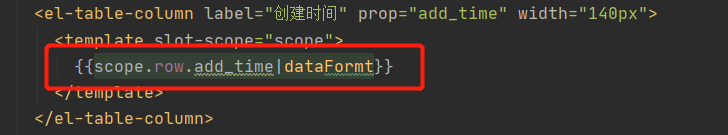# Register global time filter in main.js in Vue

In main.js, define a global time filter through: Vue. Filter().
the first parameter is the name of the filter, such as: dataform,
the second parameter is the processing function of the time filter: function(), which needs to specify a formal parameter: originval, which is to process the time data
to get the originval time, first update the date and pass the originval to you, that is, to get a date object of the time according to the local time

Get four digit year through getfullyear()

Get the month through getmonth(). The month starts from 1, so make it + 1
if the month is less than two digits, fill in 0 in the front by calling. Padstart (2, ‘0’). The first parameter represents the total length of the number of digits, and the second parameter is a string. If the number of digits is less than two, which string should be filled in

Get the current date through: getdate()

Get the current hour through: gethours()

Get the current minutes through: getminutes()

Get the current seconds by: getseconds()

Let the obtained date, time, minute and second be spliced into a complete date string
return ` \${y} - \${m} - \${D} - \${HH}: \${mm}: \${SS} `

//Format time filter
vue.filter (‘dataform ‘, function (originval) {
const DT = new date (originval)

const y = dt.getFullYear()
const m = (dt.getMonth() + 1 + ‘’).padStart(2, ‘0’)
const d = (dt.getDate() + ‘’).padStart(2, ‘0’)

const hh = (dt.getHours() + ‘’).padStart(2, ‘0’)
const mm = (dt.getMinutes() + ‘’).padStart(2, ‘0’)
const ss = (dt.getSeconds() + ‘’).padStart(2, ‘0’)

return `\${y}-\${m}-\${d} \${hh}:\${mm}:\${ss}`